Question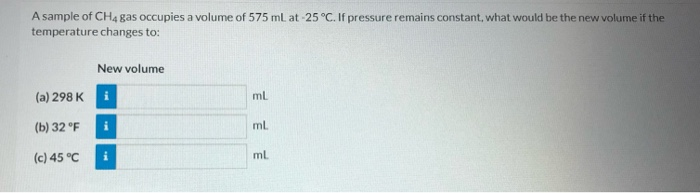We need at least 10 more requests to produce the answer.

0 / 10 have requested this problem solution

The more requests, the faster the answer.

All students who have requested the answer will be notified once they are available.

#### Earn Coins

Coins can be redeemed for fabulous gifts.

Similar Homework Help Questions
• ### 1. Hydrogen gas occupies a volume of 575 ml at 117.3 kPa and 50 °C. What will the new volume be when the pressure i...1. Hydrogen gas occupies a volume of 575 ml at 117.3 kPa and 50 °C. What will the new volume be when the pressure increases to 133.3 kPa and the temp is 45 °C?

• ### A sample of carbon monoxide gas occupies a volume of 263 mL at a pressure of...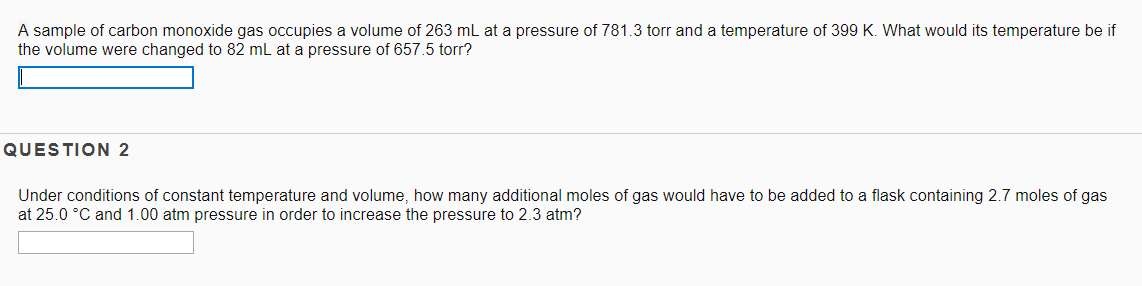A sample of carbon monoxide gas occupies a volume of 263 mL at a pressure of 781.3 torr and a temperature of 399 K. What would its temperature be if the volume were changed to 82 mL at a pressure of 657.5 torr? QUESTION 2 Under conditions of constant temperature and volume, how many additional moles of gas would have to be added to a flask containing 2.7 moles of gas at 25.0 °C and 1.00 atm pressure in order...

• ### a sample of gas at 0.75 atm occupies a volume of 521 mL. if the temperature...

a sample of gas at 0.75 atm occupies a volume of 521 mL. if the temperature remains constant, what will be the new pressure if the volume increases to 776 ml ?

• ### PRINTER VERSION Question 4 A sample of Oz gas occupies a volume of 617 mL at...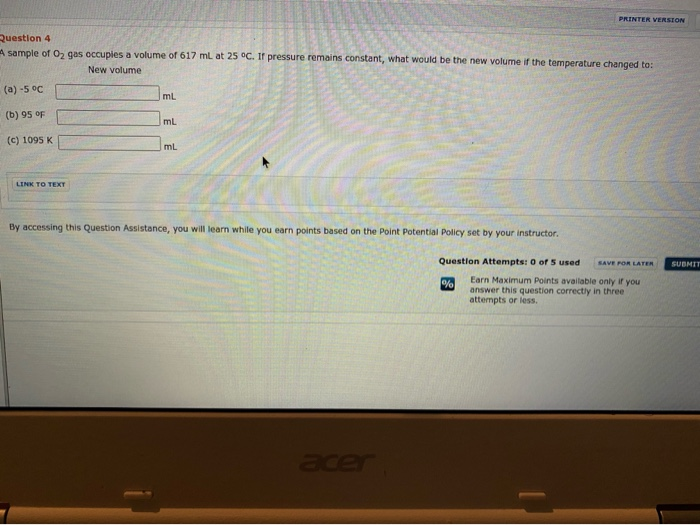PRINTER VERSION Question 4 A sample of Oz gas occupies a volume of 617 mL at 25°C. If pressure remains constant, what would be the new volume if the temperature changed to: New volume (a) -5 oC ml (b) 95 OF ml (c) 1095 K mL LINK TO TEXT By accessing this Question Assistance, you will learn while you earn points based on the Point Potential Policy set by your instructor. SUBMIT Question Attempts: 0 of 5 used SAVE POR...

• ### A sample of argon gas at a pressure of and a temperature of, occupies a volume...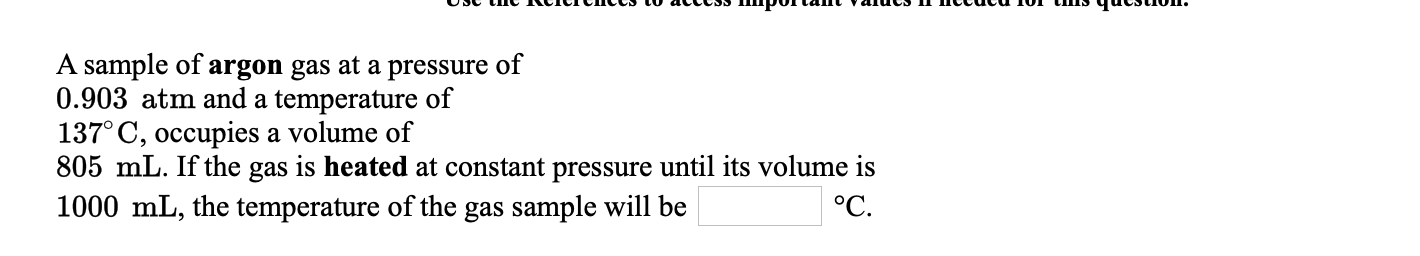A sample of argon gas at a pressure of and a temperature of, occupies a volume of. If the gas is heated at constant pressure until its volume is, the temperature of the gas sample will be  °C. от и се спес 0 accc Ппрола напис п псиса по иппо чистио. A sample of argon gas at a pressure of 0.903 atm and a temperature of 137°C, occupies a volume of 805 mL. If the gas is heated at constant pressure...

• ### A sample of oxygen gas at a pressure of and a temperature of, occupies a volume...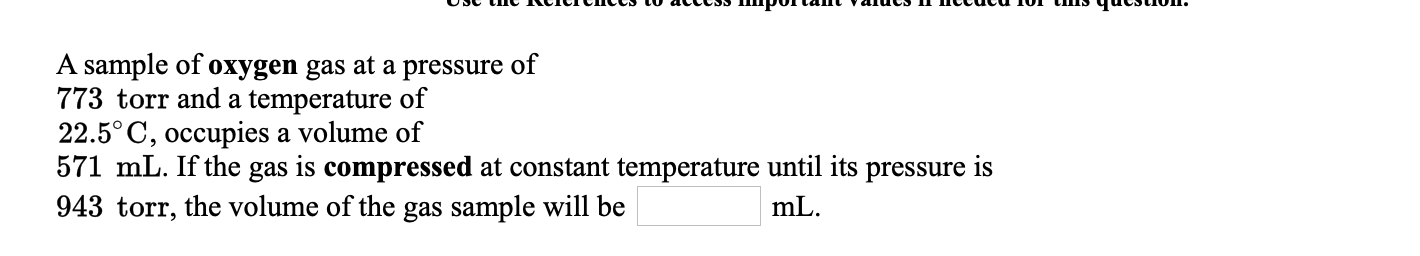A sample of oxygen gas at a pressure of and a temperature of, occupies a volume of. If the gas is compressed at constant temperature until its pressure is, the volume of the gas sample will be  mL. от и се спес 0 accc Ппрола напис п псиса по иппо чистио. A sample of oxygen gas at a pressure of 773 torr and a temperature of 22.5°C, occupies a volume of 571 mL. If the gas is compressed at constant temperature...

• ### A sample of nitrogen gas at a pressure of and a temperature of, occupies a volume...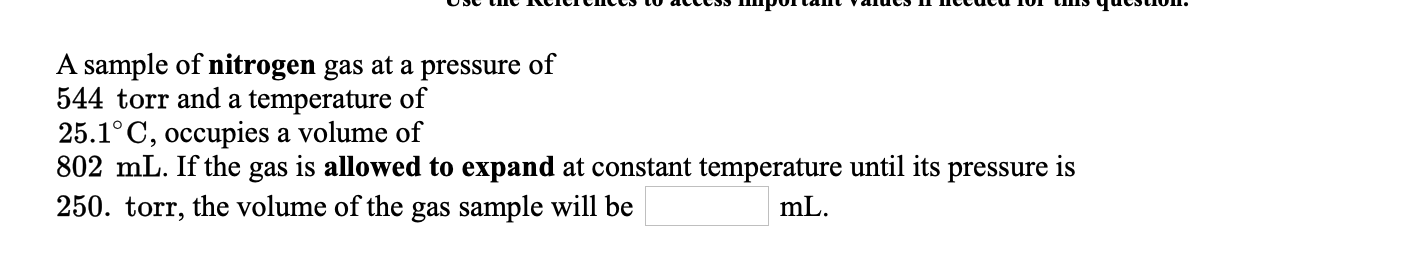A sample of nitrogen gas at a pressure of and a temperature of, occupies a volume of. If the gas is allowed to expand at constant temperature until its pressure is, the volume of the gas sample will be  mL. от и се спес 0 accc Пирота раис п пссаса ол и чистиоп. A sample of nitrogen gas at a pressure of 544 torr and a temperature of 25.1°C, occupies a volume of 802 mL. If the gas is allowed to...

• ### Please answer & explain 26-30 A gas sample is held at constant pressure. The gas occupies...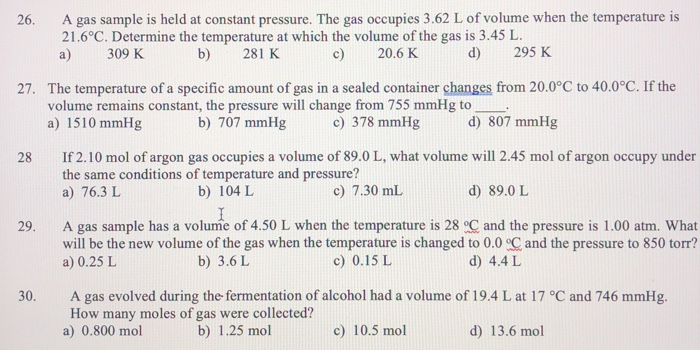Please answer & explain 26-30 A gas sample is held at constant pressure. The gas occupies 3.62 L of volume when the temperature is 21.6°C. Determine the temperature at which the volume of the gas is 3.45 L 26 d) 295 K 309 K b) 281 K c) 20.6 K a) 27. The temperature of a specific amount of gas in a sealed container changes from 20.0°C to 40.0°C. If the volume remains constant, the pressure will change from 755...

• ### 18 of 24 > A sample of xenon gas occupies a volume of 6.26 L at...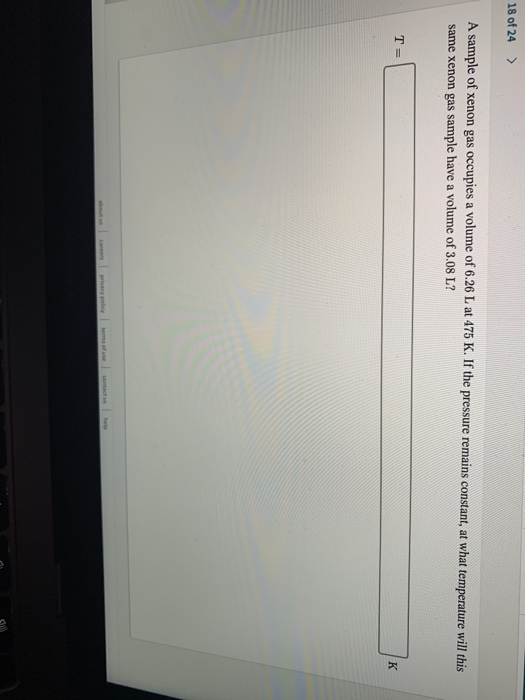18 of 24 > A sample of xenon gas occupies a volume of 6.26 L at 475 K. If the pressure remains constant, at what temperature will this same xenon gas sample have a volume of 3.08 L? TE

• ### 22. A sample of gas occupies 6.00 liters at STP. If the pressure remains constant and...

22. A sample of gas occupies 6.00 liters at STP. If the pressure remains constant and the temperature is raised to 546 oC, the volume of the gas sample would be a. 2.00 L b. 3.00 L c. 6.00 L d. 12.0 L e. 18.0 L